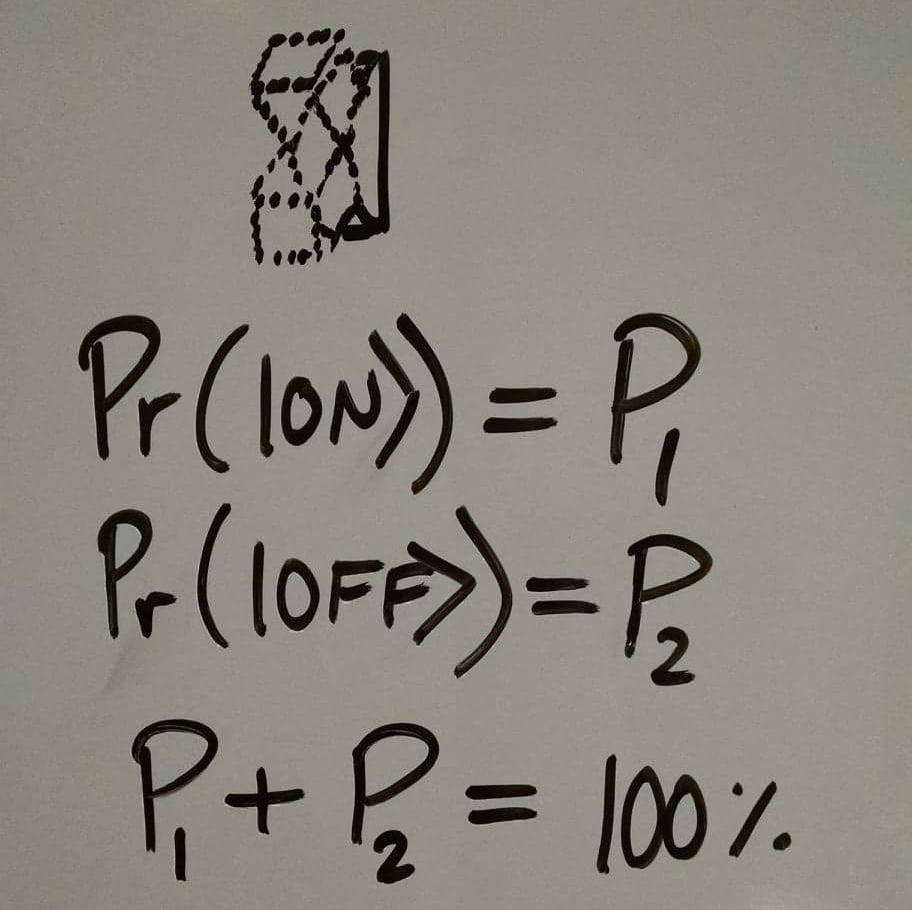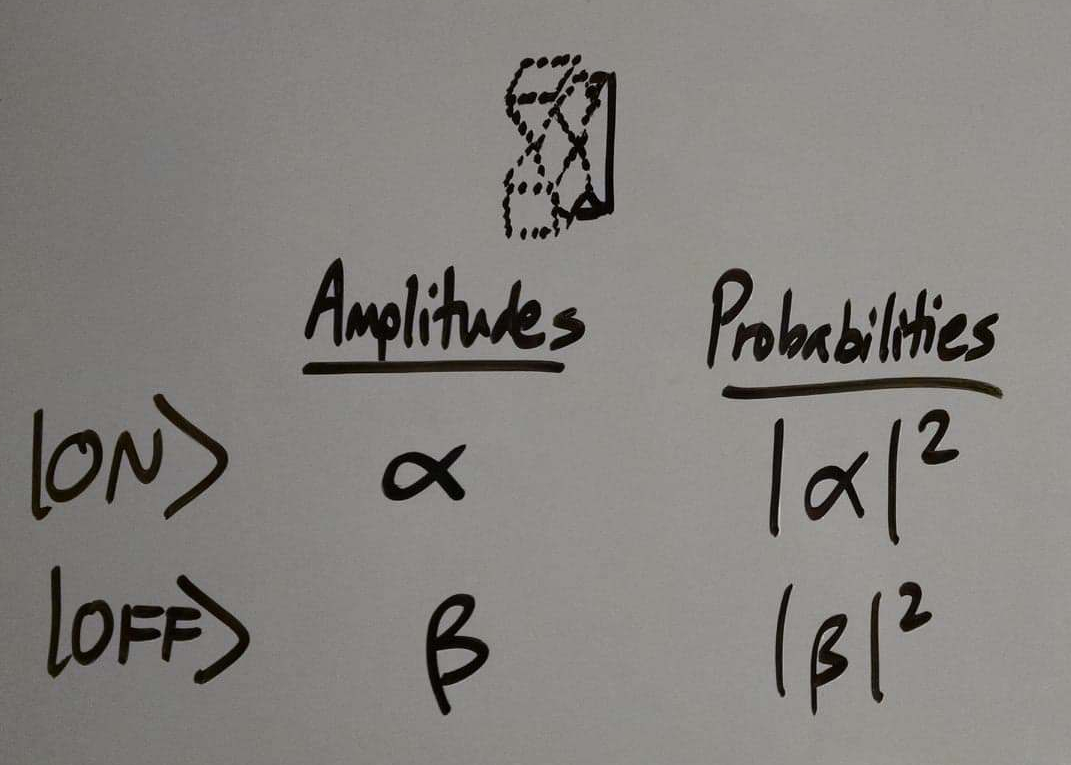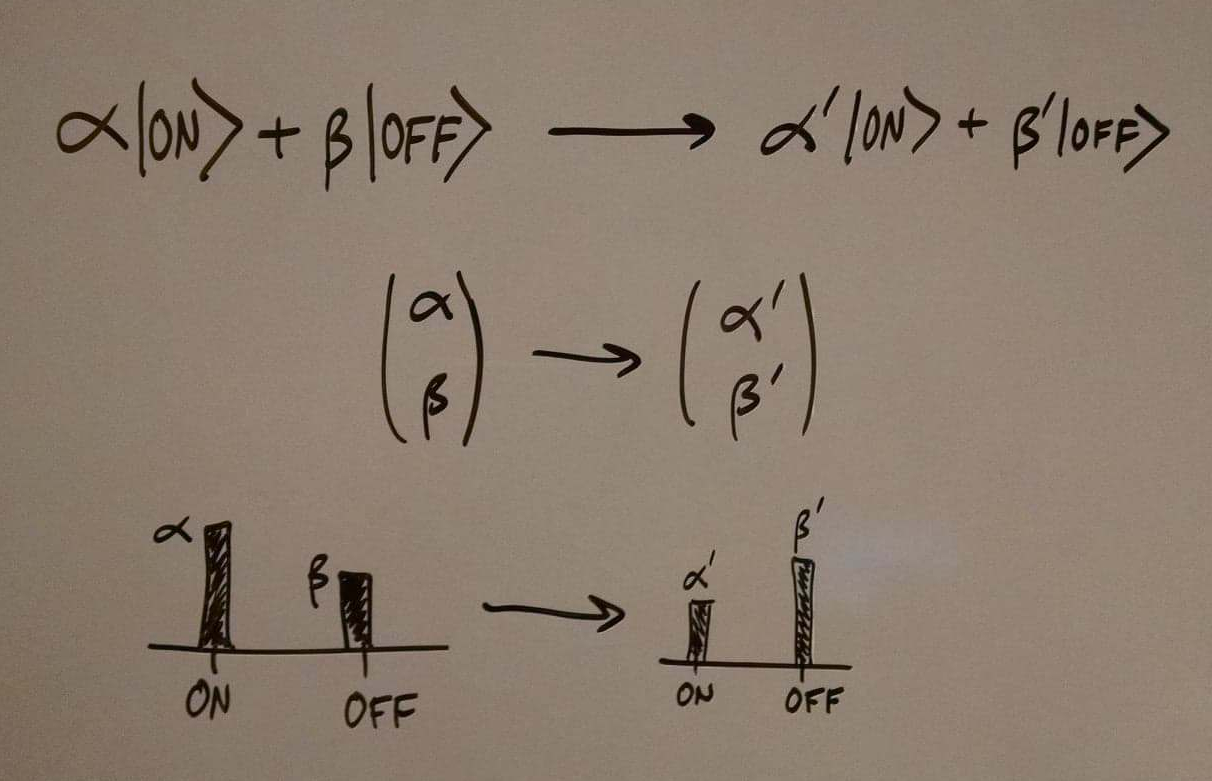# Quantum Computing in a Nutshell

You can think about classical bits as like little switches. They have two possible states, ON and OFF, and are in either one or the other.Then we have logic gates, which are like operations we perform on the switches. If we have one switch, we might flip it from whatever state it’s in to the opposite state. If we have two, we might flip the second switch conditional on the first switch being ON, and otherwise do nothing. And so on.Put together a bunch of these bits and gates and you get an algorithm. If you arrange them cleverly enough, you end up getting interesting computations like adding binary numbers and factoring primes and playing Skyrim.

Quantum computing is a fundamentally different beast. To start with, the fundamental units of quantum computation are non-deterministic. We call them qubits, for quantum bits. While a qubit can, like a classical bit, be in the state ON and the state OFF, it can also be in other more exotic states:Important! Just like a classical bit, a qubit will only ever be observed in two possible states, ON and OFF. We never observe a qubit being in the state “36% ON and 64% OFF.” But if we prepare a qubit in that particular state, and then measure it over and over again, we could find that 36% of the time it is ON and 64% of the time it is OFF. That is, the state of a qubit specifies the probability distribution over possible observed outcomes.

Let’s compare the complexity of bits to the complexity of qubits.

To specify the state of a classical bit, we only need to answer a single yes or no question: Is it ON?

To specify the state of a quantum bit, we need to answer a more complicated question: What is the probability that it is ON?

Since probabilities are real numbers, answering the second question requires infinitely more information than answering the first. (In general, specifying a real number to perfect precision requires answering an unbounded number of yes or no questions.) The implication of this is that in some sense, the state of a qubit contains infinitely more information than the state of a classical bit. The trick of quantum computing is to exploit this enhanced information capacity in order to build quantum algorithms that beat out the best classical algorithms.

Moving on! The set of all states that a qubit can be in is the set of all probability distributions over the outcomes ON and OFF.Now, we could describe a simple probabilistic extension to classical computation, where logic gates transform probability distributions into different probability distributions, and algorithms neatly combine these gates to get useful computations, and be done with it. But it turns out that things are a slight bit more complicated than this. Having described this probabilistic extension, we would still not have quantum computing.

In fact, the states of qubits are specified not by probability distributions over the observations ON and OFF, but an amplitude distribution over these observations.

What are these amplitudes? An amplitude is simply a thing that you square to get the probability of the observation. Amplitudes have a wider range of possible values than probabilities. While a probability has to be greater than zero, an amplitude can be negative (since the square of a negative number will still be positive). In fact, amplitudes can even be complex numbers, in which case the square of the amplitude is just the square of its distance from zero in the complex plane.Now, why does it matter that the set of quantum states be described by amplitudes rather than probabilities? After all, the amplitudes will always just be converted back to probabilities when we observe the qubits, so what difference does it make if the probability came from a negative amplitude or a positive one? The answer to this question is difficult, but it comes down to this:

For some reason, it appears that on the quantum level, the universe calculates the states of particles in terms of these complex numbers we call amplitudes, not probabilities. This was the discovery of experimentalists in the 1900s that looked at things like the double slit experiment, the Stern-Gerlach experiment, and so on. If you try to just analyze everything in terms of probabilities instead of amplitudes, you will get the wrong answer.

We’ll write the state of a qubit that has an amplitude alpha of being ON and an amplitude beta of being OFF as follows:It’s useful to have a few different ways of visualizing the state of a qubit.Now, quantum gates are transformations that take amplitude distributions to different amplitude distributions.The set of physically realizable quantum gates is the set of all transformations that take normalized states (|α|² + |β|² = 1) to other normalized states. Some of these gates are given special names like the Hadamard gate or the CNOT gate and crop up all the time. And just like with classical states, you can put together these gates in clever ways to construct algorithms that do interesting computations for you.

So, that’s quantum computing!

Now, the first thing to notice is that the set of all things that quantum computers can do must contain the set of all things that classical computers can do. Why? Because classical computers are just a special case of quantum computers where states are only allowed to be either ON or OFF. Every classical logic gate has a parallel quantum logic gate, and so every classical algorithm is a quantum algorithm is disguise.

But quantum computers can also do more than classical computers. In the next posts, I’ll give two examples of quantum algorithms we’ve discovered that solve problems at speeds that are classically impossible: the Deutsch-Jozsa algorithm and Grover’s algorithm. Stay tuned!

(Next: More on quantum gates)